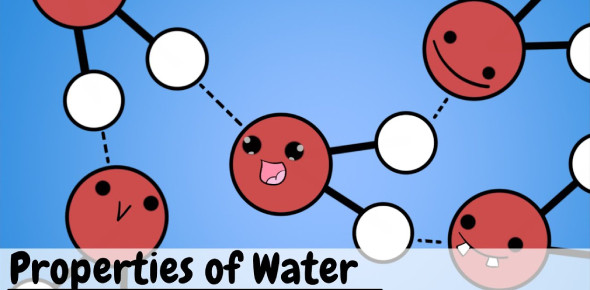# Water Properties Trivia! Chemistry Practice Quiz

27 Questions | Total Attempts: 902SettingsDo you know anything about water properties? Water is a polar inorganic combination that is a tasteless, odorless liquid. It is the most studied chemical compound. It is also the most abundant substance on earth. Water has the chemical formula of H20. It is one molecule of water, two hydrogen atoms bonded to a single oxygen atom. See how much you know about the properties of water and try this quiz.

• 1.
Which of the following things decrease as you descend into the ocean?
• A.

Density

• B.

Pressure

• C.

Salinity

• D.

Gasses(oxygen and carbon dioxide)

• E.

Temperature

• F.

Light

• 2.
Sodium chloride is the scientific name for _______________________
• 3.
We can calculate the density of something by
• A.

Weighing it

• B.

Dividing the volume by the mass

• C.

Multiplying the volume by the mass

• D.

Dividing the mass by the volume

• E.

Multiplying the mass by the volume

• F.

Placing it in water

• 4.
Water evaporating from the ocean would decrease the salinity of the water.
• A.

True

• B.

False

• 5.
The water around the mouth of a river will have a higher level of salinity.
• A.

True

• B.

False

• 6.
When water from the ocean freezes near the poles the ocean salinity will increase.
• A.

True

• B.

False

• 7.
Mr. Pautler had to move classrooms so he got this huge box that was 10ft2.  When he filled it with books it weighed 70 pounds.  Now, this was no problem for him to carry, but as he is a scientist he was wondering what the density of the box would be.  The density of the box would be _______________ lbs/ft2
• 8.
As you descend into the ocean the density of the water increases.
• A.

True

• B.

False

• 9.
As you descend into the ocean the salinity of the water increases.
• A.

True

• B.

False

• 10.
As you descend into the ocean the temperature of the water increases.
• A.

True

• B.

False

• 11.
As you descend into the ocean the amount of light in the water increases.
• A.

True

• B.

False

• 12.
Is hot or cold water denser?
• A.

Hot

• B.

Cold

• C.

Neither(they are the same)

• 13.
Is salt water or fresh water denser?
• A.

Salt

• B.

Fresh

• C.

Neither(they are the same)

• 14.
You have a mystery item that you put into a tub of water.  The mystery item floats in the water.  You now know that the mystery item is _____________________ than water.
• A.

More dense

• B.

Less dense

• C.

Higher in salinity

• D.

Lower in salinity

• 15.
The Justin Bieber doll weighs 10 ounces and it takes up 100 cubic centimeters.  It's density is ____________
• A.

10 ounces per cubic centimeter

• B.

1 ounce per cubic centimeter

• C.

1000 ounces per cubic centimeter

• D.

.1 ounce per cubic centimeter

• 16.
Salt in water does all of the following except for
• A.

It lowers the freezing temperature of the water

• B.

It increases its buoyancy

• C.

It increases its density

• D.

It increases its volume

• 17.
_______________________________ is the weight of all the water above you pressing on you in all directions.
• 18.
There is a small amount of light in the transition zone.
• A.

True

• B.

False

• 19.
A diver may get "the Bends" if which type of gas forms bubbles in their blood when swimming to the top too quickly.
• A.

Oxygen

• B.

Carbon Dioxide

• C.

Nitrogen

• D.

Helium

• 20.
If you increase the volume of something but keep its mass the same, what are you doing to the density?
• A.

Increasing it

• B.

Decreasing it

• C.

Keeping it the same.

• 21.
A good synonym for volume is
• A.

Weight

• B.

Distance

• C.

Thickness

• D.

Size

• E.

Compactness

• 22.
A good synonym for mass is
• A.

Weight

• B.

Volume

• C.

Thickness

• D.

Size

• E.

Compactness

• 23.
What part of ocean water is salt?
• A.

35%

• B.

35 parts per thousand

• C.

.35%

• D.

3.5 parts per thousand

• 24.
Salt water needs a lower temperature to freeze.
• A.

True

• B.

False

• 25.
There are a few reasons why an area may have a high salinity.  Which is the main reason that the Dead sea has this situation?
• A.

Evaporation

• B.

Precipitation

• C.

Freezing

• D.

Depth of the water

Related TopicsBack to top